# Hyperbolic Functions: Definition & Example

Lesson Transcript
Instructor
Russell Frith
Expert Contributor
Robert Ferdinand

Robert Ferdinand has taught university-level mathematics, statistics and computer science from freshmen to senior level. Robert has a PhD in Applied Mathematics.

Hyperbolic functions are named the same as trigonometric functions with the letter 'h' added to each name. In this lesson, definitions and examples are introduced. Updated: 06/02/2020

## Hyperbolic Functions

If you work in engineering or physics, you have to work with a lot of models. Well, to solve modeling problems you'll need hyperbolic functions.

Certain even and odd combinations of the exponential functions ex and e-x arise so frequently in engineering, physics, and mathematics that they're given special names. Hyperbolic functions are analogous trigonometric functions in that they are named the same as trigonometric functions with the letter 'h' appended to each name.

These special functions have the same relationship to the hyperbola that trigonometric functions have to the circle. For this reason, they are collectively known as hyperbolic functions and are individually called hyperbolic sine, hyperbolic cosine, and so on. In addition to modeling, they can be used as solutions to some types of partial differential equations.

Think of taking a rope, fixing the two ends, and then letting it hang under the force of gravity. The shape of the rope will naturally form a hyperbolic cosine curve.An error occurred trying to load this video.

Try refreshing the page, or contact customer support.

Coming up next: Cauchy-Riemann Equations: Definition & Examples

### You're on a roll. Keep up the good work!

Replay
Your next lesson will play in 10 seconds
• 0:04 Hyperbolic Functions
• 1:04 Types & Graphs
• 4:19 Examples
• 6:13 The List of Properties
• 7:01 Lesson Summary
Save Save

Want to watch this again later?

Timeline
Autoplay
Autoplay
Speed Speed

## Types & Graphs

#### Hyperbolic Sine: y = sinh(x)

This math statement is read as 'y equals hyperbolic sine x' or 'sinch x.'

The exponential formula is: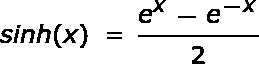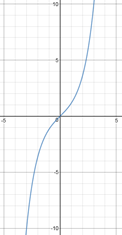Note that the domain and range of this function are both the set of real numbers. This function is also one-to-one.

#### Hyperbolic Cosine: y = cosh(x)

This math statement is read as 'y equals hyperbolic cosine x' or 'cosh x.'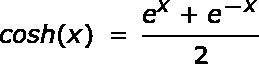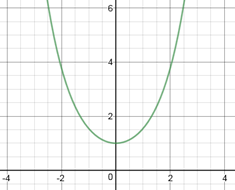Note that the graph is symmetric with respect to the y-axis and it has a minimum vertex at (0, 1). The domain of this function is the set of real numbers and the range is any number equal to or greater than one.

#### Hyperbolic Tangent: y = tanh(x)

This math statement is read as 'y equals hyperbolic tangent x' or 'tanch x.'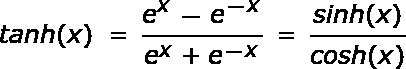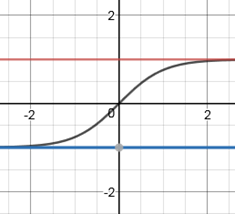Note that the graph is symmetric with respect to the origin. The lines y = 1 and y = -1 are both horizontal asymptotes. The domain of the function is the set of real numbers. The range is the open interval (-1, 1).

#### Hyperbolic Cotangent: y = coth(x)

This math statement is read as 'y equals hyperbolic cotangent x' or 'coth x.'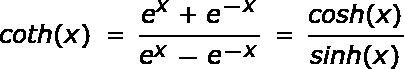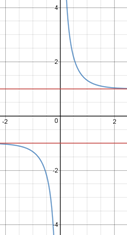Note that the graph is symmetric with respect to the origin. The lines y = 1 and y = -1 are both horizontal asymptotes. The y-axis is a vertical asymptote. The domain of the function is the set of real numbers excluding zero. The range is (-infinity, -1) union (1, infinity).

#### Hyperbolic Secant: y = sech(x)

This math statement is read as 'y equals hyperbolic secant x.'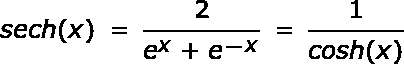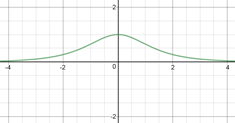The x-axis is a horizontal asymptote. The graph is symmetric with respect to the y-axis. The domain of the function is the set of real numbers. The range of the function is the half-open interval (0, 1].

#### Hyperbolic Cosecant: y = csch(x)

This math statement is read as 'y equals hyperbolic cosecant x.'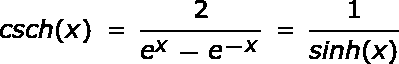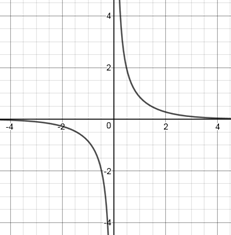Note that both the x- and y-axes are asymptotes. The graph is symmetric with respect to the origin. Both the domain and range of the function are the set of real numbers excluding zero.

To unlock this lesson you must be a Study.com Member.

## Hyperbolic Trigonometric Functions: Practice Problems

#### Key Terms

• Hyperbolic Trigonometric Functions: Functions related to Hyperbolas

• Paper
• Pencil
• Calculator

### Practice Problems (Show all your work)

(a) Find all six hyperbolic trigonometric function values for the angle 60 degrees

(a) First, we need to convert our angle from degrees to radians as follows:

x = 60 degrees = 60 x pi/180 = pi/3 radians.

Next, we use the radian measure and a calculator to calculate the six hyperbolic trigonometric function values for our angle of x = pi/3 radians:

• sinh (pi/3) = (e(pi/3) - e(-pi/3)) / 2 = 1.25.

• cosh (pi/3) = (e(pi/3) + e(-pi/3)) / 2 = 1.60.

• tanh (pi/3) = (e(pi/3) - e(-pi/3)) / (e(pi/3) + e(-pi/3)) = 0.78.

• coth (pi/3) = (e(pi/3) + e(-pi/3)) / (e(pi/3) - e(-pi/3)) = 1.28.

• csch (pi/3) = 2/ (e(pi/3) - e(-pi/3)) = 0.80.

• sech (pi/3) = 2/(e(pi/3) + e(-pi/3)) = 0.63.

### Register to view this lesson

Are you a student or a teacher?